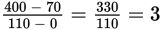# SAT Math Multiple Choice Question 388: Answer and Explanation

### Test Information

Question: 388

13. Lena bought a saltwater fish tank that holds 400 gallons of water. She started filling the tank on Friday, but then stopped after putting only 70 gallons of water in the tank. On Saturday, she bought a bigger hose and began filling the tank again. It took her 1 hour and 50 minutes on Saturday to completely fill the tank. Which equation represents the number of gallons of water in the fish tank on Saturday, given the amount of time in minutes that Lena spent filling the tank?

• A. y = 3x + 70
• B. y = 3x + 330
• C. y = 70x + 330
• D. y = 110x + 70

Explanation:

A

Difficulty: Hard

Category: Heart of Algebra / Linear Equations

Strategic Advice: To write the equation of a line, you need two things—the starting amount (y-intercept) and the rate of change (slope). Substitute these values into slope-intercept form of a line (y = mx + b), and you have your equation.

Getting to the Answer: The initial amount of water in the tank on Saturday is 70 gallons, so you already know b. To find m, you'll need to use the information given in the question to write two data points.

The amount of water in the tank depends on how long Lena has been filling it, so the number of gallons is the dependent variable and time is the independent variable. This tells you that the data points should be written in the form (time, gallons). At time = 0 on Saturday, the number of gallons is 70, so the data point is (0, 70). After 1 hour and 50 minutes, which is 60 + 50 = 110 minutes, the tank is full (400 gallons), so another data point is (110, 400). Now, use the slope formula to find that the rate of change isgallons per minute. Substituting m and b into slope-intercept form results in the equation y = 3x + 70, so (A) is correct.